Copyright (C) 2012-present, The Computational Data Science Lab
https://github.com/cdslaborg/paramonte

# Making histfit plots with the ParaMonte visualization tools

NOTE
If you are viewing an HTML version of this MATLAB live script on the web, you can download the corresponding MATLAB live script visualization_histfit.mlx file to this HTML page at,
https://github.com/cdslaborg/paramontex/tree/main/MATLAB/mlx
Once you download the file, open it in MATLAB to view and interact with its contents, which is the same as what you see on this page.
First, let's clean up the MATLAB environment and make sure the path to the ParaMonte library is in MATLAB's path list.
clc;
clear all;
close all;
format compact; format long;
%%%%%%%%%%%% IMPORTANT %%%%%%%%%%%%%
% Set the path to the ParaMonte library:
% Change the following path to the ParaMonte library root directory,
% otherwise, make sure the path to the ParaMonte library is already added
% to MATLAB's path list.
pmlibRootDir = './';
%%%%%%%%%%%%%%%%%%%%%%%%%%%%%%%%%%%%
% change MATLAB's working directory to the folder containing this script
% if MATLAB Live Scripts did not create a temporary folder, we would not
% have all of these problems!
try
setwdFilePath = websave("setwd.m","https://github.com/cdslaborg/paramontex/raw/main/MATLAB/mlx/setwd.m");
run(setwdFilePath); % This is a MATLAB script that you can download from the same GitHub location given in the above.
catch % alas, we will have to run the simulations in MATLAB Live Script's temporary folder
filePath = mfilename('fullpath');
[currentDir,fileName,fileExt] = fileparts(filePath); cd(currentDir);
cd(fileparts(mfilename('fullpath'))); % Change working directory to source code directory.
end

## ParaMonte's default visualization tools

The ParaMonte library ships with several visualization tools that automate much of the MATLAB coding required to visualize the output of the simulations performed by the ParMonte library samplers.
By replacing the input dataFrame to these tools and following the conventions of the ParaMonte library, one can also use these visualization tools for any dataset that may not have been generated by the ParaMonte library.
Consider the following refined Markov chain sample on the web generated by the ParaDRAM sampler of the ParaMonte library to sample a MultiVariate Normal distribution. Since this is a sample file as inidicated by its suffix "_sample.txt" We will read this file via the ParaDRAM sampler's readSample() method.
pm = paramonte();
This method automatically generates a set of tools that can be used to visualize the contents of the compact chain file. Note that these visualization tools are not unique to this particular method of the ParaDRAM sampler or other ParaMonte samplers. For the sake of illustration however, we will create plots using the above dataset read via readChain() method of the ParaDRAM routine.
chain = pmpd.sampleList{1};
chain.plot.histfit.make();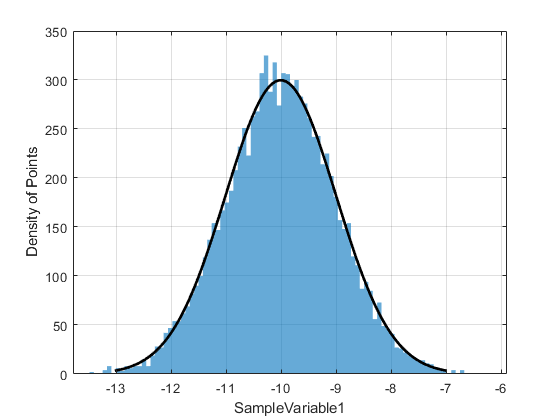To draw the a histogram and then fit the histogram by a distribution, the ParaMonte visualizer utilizes the histfit() function of MATLAB. One can a limited number of the properties passed to this MATLAB function such as nbins and dist via,
chain.plot.histfit.histfit
ans = struct with fields:
enabled: 1 dist: "Normal" nbins: []
chain.plot.histfit.histfit.nbins = 20; % add a new histfit attribute
chain.plot.histfit.make();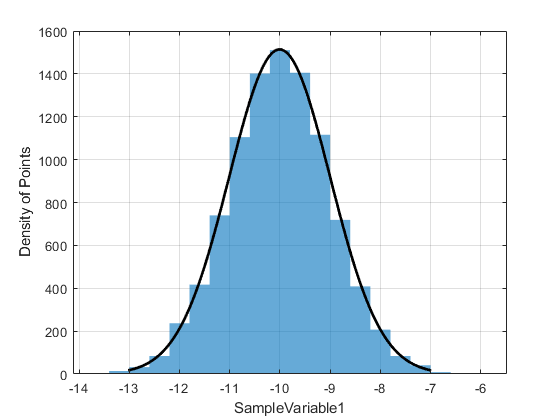To reset the properties of the plot object to the default settings, try,
chain.plot.histfit.reset();
ParaDRAM - NOTE: resetting the properties of the histfit plot...
To reset the entire plot object including reading the data again from the input dataFrame, try,
chain.plot.histfit.reset("hard");
ParaDRAM - NOTE: creating the histfit plot object from scratch...
Remember that a handle to all objects in the plot is also stored in the currentFig component of the object. Most of the properties of the figure, axes, and the plots can be also changed directly via these handles. For example, to change the colorbar label, we could try,
chain.plot.histfit.make();chain.plot.histfit.currentFig.histfit{1}
ans =
2×1 graphics array: Bar Line
chain.plot.histfit.make();
chain.plot.histfit.currentFig.histfit{1}(1).FaceColor = "red"; % make histogram color red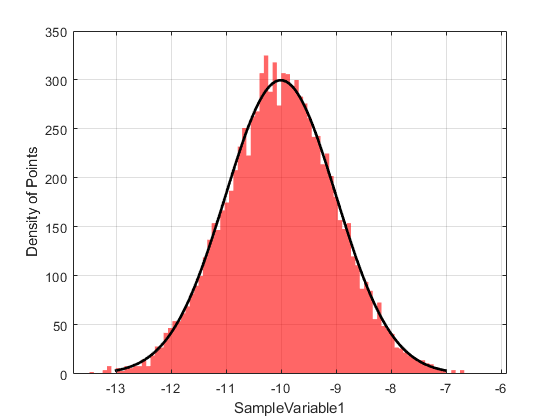## Choosing different or multiple columns of data to plot

By default, the column named the first two variables of the sampled space are shown in the plot. This can be readily changed to any paris of variables, like,
chain.df.Properties.VariableNames
ans = 1×5 cell array {'SampleLogFunc'} {'SampleVariable1'} {'SampleVariable2'} {'SampleVariable3'} {'SampleVariable4'}
chain.plot.histfit.reset();
ParaDRAM - NOTE: resetting the properties of the histfit plot...
chain.plot.histfit.make( "xcolumns", ["SampleVariable1", 3:5]);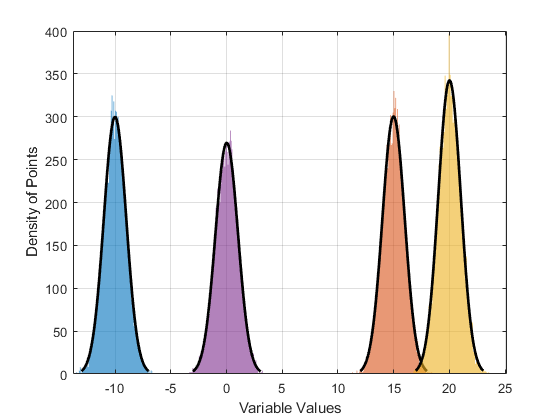Notice the possibility of use of both column indices and column names to point to a data column in the dataFrame.
Perhaps we would also want the legends,
chain.plot.histfit.legend.enabled = true;
chain.plot.histfit.legend.kws.location = "northwest";
chain.plot.histfit.make( "xcolumns", ["SampleVariable1", 3:5]);
ylim([0, 380]); % increase the y range to create room for the legend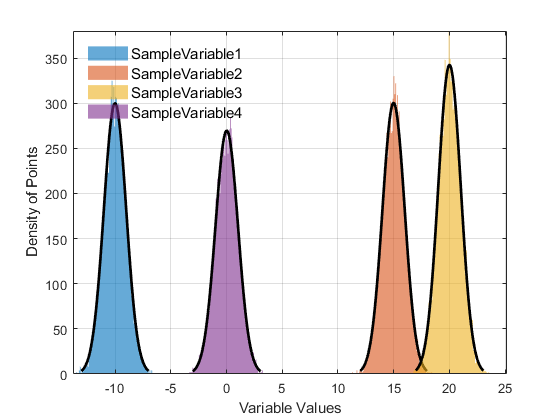To change the labels to something other than the data column names, try,
chain.plot.histfit.legend.labels = ["Variable 1","Variable 2","Variable 3","Variable 4"];
chain.plot.histfit.make( "xcolumns", ["SampleVariable1", 3:5]);
ylim([0, 380]); % increase the y range to create room for the legend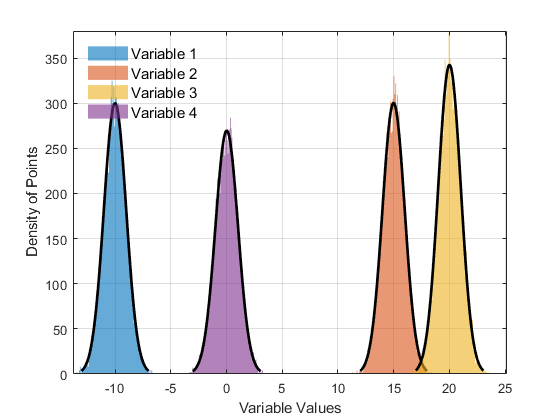## Plotting specific rows of data

Selected rows of data can be also plotted, if not all data observations have to be included. For example, we can jump through the sampled points to include only a select number of states in the plot,
chain.plot.histfit.reset();
ParaDRAM - NOTE: resetting the properties of the histfit plot...
chain.plot.histfit.rows = 1:30:chain.count; % plot every one out of 10 data rows, starting from the the first state to the end of the chain.
chain.plot.histfit.make("xcolumns", 2:5);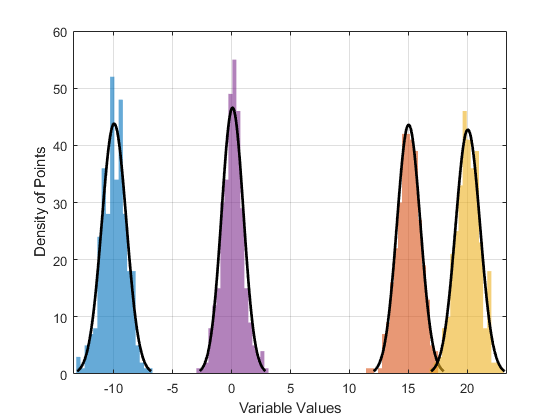## Adding targets to the plot

One can also add target values to the plots, use the target() component of the plot object. For example, one may want to add a horizontal line as the target, representing for example the mean and the standard deviation of the sample,
chain.plot.histfit.reset();
ParaDRAM - NOTE: resetting the properties of the histfit plot...
data.avg = mean(chain.df.SampleVariable1);
data.std = std(chain.df.SampleVariable1);
chain.plot.histfit.make();
chain.plot.histfit.target.vline.kws.linewidth = 1.5;
chain.plot.histfit.target.make("values", [ data.avg, nan ] ); % vertical line representing the mean of data
chain.plot.histfit.target.vline.kws.linestyle = ":";
chain.plot.histfit.target.make("values", [ data.avg + data.std, nan ... % horizontal line representing the 1-sigma upper limit of data
; data.avg - data.std, nan ... % horizontal line representing the 1-sigma lower limit of data
] ...
);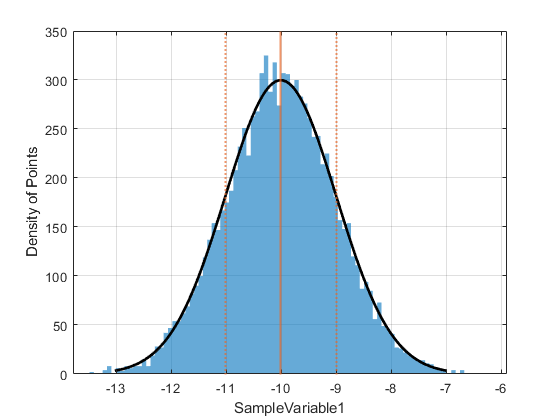In the above example, each row of the input values represents a separate target to be added to the plot. By convension, if any component of the input values is nan, that target corresponding to that component will not be added to the plot. For example,
chain.plot.histfit.target.make( "values", [ data.avg, nan ] );
is equivalent to,
chain.plot.histfit.target.hline.enabled = false;
chain.plot.histfit.target.scatter.enabled = false;
chain.plot.histfit.target.make( "values", [ data.avg, 0 ] ); % or any value other than zero, which will be ignored anyway.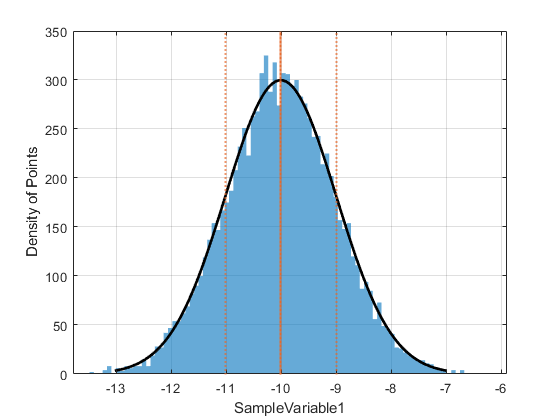## Exporting figures to external files

To extract a figure to an external PNG file, try,
chain.plot.histfit.exportFig("exportedFigure.png","-m4");The above command will extract the current active figure to an output file with the relatively high resoluton as specified by the flag -m4. To make the exported figure smaller, one could specify -m2 instead. In addition, to generate a figures with background transparency, the flag -transparent can be added to the exportFig function call,
% chain.plot.histfit.exportFig("exportedFigure.png","-m2 -transparent") % uncomment to export the figure with transparency
Final Note:
To see other more sophisticated types of plots that can be automatically made with the ParaMonte visualization tools, visit: https://www.cdslab.org/paramonte/notes/examples/matlab/mlx/

{% comment %}
{% endcomment %}